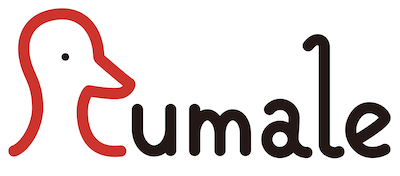# RumaleRumale (*Ru*by *ma*chine *le*arning) is a machine learning library in Ruby. Rumale provides machine learning algorithms with interfaces similar to Scikit-Learn in Python. Rumale supports Support Vector Machine, Logistic Regression, Ridge, Lasso, Multi-layer Perceptron, Naive Bayes, Decision Tree, Gradient Tree Boosting, Random Forest, K-Means, Gaussian Mixture Model, DBSCAN, Spectral Clustering, Mutidimensional Scaling, t-SNE, Fisher Discriminant Analysis, Neighbourhood Component Analysis, Principal Component Analysis, Non-negative Matrix Factorization, and many other algorithms.

## Installation

``````gem 'rumale'
``````

And then execute:

``````\$ bundle
``````

Or install it yourself as:

``````\$ gem install rumale
``````

## Usage

### Example 1. Pendigits dataset classification

``````\$ wget https://www.csie.ntu.edu.tw/~cjlin/libsvmtools/datasets/multiclass/pendigits
\$ wget https://www.csie.ntu.edu.tw/~cjlin/libsvmtools/datasets/multiclass/pendigits.t
``````

Training of the classifier with Linear SVM and RBF kernel feature map is the following code.

``````require 'rumale'

# Map training data to RBF kernel feature space.
transformer = Rumale::KernelApproximation::RBF.new(gamma: 0.0001, n_components: 1024, random_seed: 1)
transformed = transformer.fit_transform(samples)

# Train linear SVM classifier.
classifier = Rumale::LinearModel::SVC.new(reg_param: 0.0001, random_seed: 1)
classifier.fit(transformed, labels)

# Save the model.
File.open('transformer.dat', 'wb') { |f| f.write(Marshal.dump(transformer)) }
File.open('classifier.dat', 'wb') { |f| f.write(Marshal.dump(classifier)) }
``````

Classifying testing data with the trained classifier is the following code.

``````require 'rumale'

# Map testing data to RBF kernel feature space.
transformed = transformer.transform(samples)

# Classify the testing data and evaluate prediction results.
puts("Accuracy: %.1f%%" % (100.0 * classifier.score(transformed, labels)))

# Other evaluating approach
# results = classifier.predict(transformed)
# evaluator = Rumale::EvaluationMeasure::Accuracy.new
# puts("Accuracy: %.1f%%" % (100.0 * evaluator.score(results, labels)))
``````

Execution of the above scripts result in the following.

``````\$ ruby train.rb
\$ ruby test.rb
Accuracy: 98.7%
``````

### Example 2. Cross-validation

``````require 'rumale'

# Define the estimator to be evaluated.
lr = Rumale::LinearModel::LogisticRegression.new(learning_rate: 0.00001, reg_param: 0.0001, random_seed: 1)

# Define the evaluation measure, splitting strategy, and cross validation.
ev = Rumale::EvaluationMeasure::LogLoss.new
kf = Rumale::ModelSelection::StratifiedKFold.new(n_splits: 5, shuffle: true, random_seed: 1)
cv = Rumale::ModelSelection::CrossValidation.new(estimator: lr, splitter: kf, evaluator: ev)

# Perform 5-cross validation.
report = cv.perform(samples, labels)

# Output result.
mean_logloss = report[:test_score].inject(:+) / kf.n_splits
puts("5-CV mean log-loss: %.3f" % mean_logloss)
``````

Execution of the above scripts result in the following.

``````\$ ruby cross_validation.rb
5-CV mean log-loss: 0.355
``````

### Example 3. Pipeline

``````require 'rumale'

# Construct pipeline with kernel approximation and SVC.
rbf = Rumale::KernelApproximation::RBF.new(gamma: 0.0001, n_components: 800, random_seed: 1)
svc = Rumale::LinearModel::SVC.new(reg_param: 0.0001, random_seed: 1)
pipeline = Rumale::Pipeline::Pipeline.new(steps: { trns: rbf, clsf: svc })

# Define the splitting strategy and cross validation.
kf = Rumale::ModelSelection::StratifiedKFold.new(n_splits: 5, shuffle: true, random_seed: 1)
cv = Rumale::ModelSelection::CrossValidation.new(estimator: pipeline, splitter: kf)

# Perform 5-cross validation.
report = cv.perform(samples, labels)

# Output result.
mean_accuracy = report[:test_score].inject(:+) / kf.n_splits
puts("5-CV mean accuracy: %.1f %%" % (mean_accuracy * 100.0))
``````

Execution of the above scripts result in the following.

``````\$ ruby pipeline.rb
5-CV mean accuracy: 99.6 %
``````

## Speed up

### Numo::Linalg

Rumale uses Numo::NArray for typed arrays. Loading the Numo::Linalg allows to perform matrix product of Numo::NArray using BLAS libraries. For example, using the OpenBLAS speeds up many estimators in Rumale.

Install OpenBLAS library.

Mac:

``````\$ brew install openblas
``````

Ubuntu:

``````\$ sudo apt-get install gcc gfortran
\$ wget https://github.com/xianyi/OpenBLAS/archive/v0.3.5.tar.gz
\$ tar xzf v0.3.5.tar.gz
\$ cd OpenBLAS-0.3.5
\$ make USE_OPENMP=1
\$ sudo make PREFIX=/usr/local install
``````

Install Numo::Linalg gem.

``````\$ gem install numo-linalg
``````

In ruby script, you only need to require the autoloader module of Numo::Linalg.

``````require 'numo/linalg/autoloader'
require 'rumale'
``````

### Parallel

Several estimators in Rumale support parallel processing. Parallel processing in Rumale is realized by Parallel gem, so install and load it.

``````\$ gem install parallel
``````
``````require 'parallel'
require 'rumale'
``````

Estimators that support parallel processing have n_jobs parameter. When -1 is given to n_jobs parameter, all processors are used.

``````estimator = Rumale::Ensemble::RandomForestClassifier.new(n_jobs: -1, random_seed: 1)
``````

## Contributing

Bug reports and pull requests are welcome on GitHub at github.com/yoshoku/rumale. This project is intended to be a safe, welcoming space for collaboration, and contributors are expected to adhere to the Contributor Covenant code of conduct.